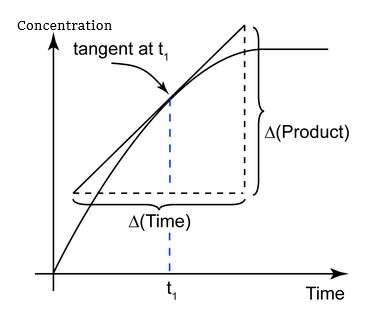# What Is Instantaneous Rate Of Reaction? How Is It Determined?

The rate of reaction or reaction rate is the speed at which reactants are converted into products.

### Instantaneous Rate Of Reaction

The instantaneous rate of reaction is defined as the change in concentration of an infinitely small time interval. An instantaneous rate is a rate at some instant in time. The average rate is the average of the instantaneous reaction rate over a period of time during the reaction. The instantaneous rate of reaction is the rate at which the reaction is proceeding at any given time.

### Expression of  Instantaneous Rate Of Reaction

Suppose the value of the term Δt is very small and tends to zero. Now, we have an infinitesimally small Δt which is a very small time period and can be considered a particular instant of time. The average reaction rate will be the instantaneous rate of reaction.

Mathematically,

Average Rate of Reaction = −Δ[A]Δt=Δ[B]Δt

When Δt →0

Instantaneous Rate of Reaction = −Δ[A]dt=Δ[B]dt

Instantaneous Rate of Reaction = −d[A]dt=d[B]dt

The unit of rate of reaction is given by concentration/time that is (mol/L)/sec.

### Determination of  Instantaneous Rate Of Reaction

We determine an instantaneous rate at time t: by calculating the negative of slope of the curve of concentration of a reactant versus time at time t. It can be measured by calculating the slope of the tangent to the graph of concentration vs time.

There are two ways to determine the instantaneous reaction rate:

• By using data from experiments and finding the slope of the tangent from the concentration-time graph.
• By using data from experiments by getting their average rate and then determining the reaction rate from the slope of the tangents.

Consider a concentration-time graph for the reaction with the P product.

The instantaneous rate of reaction is obtained from the slope of the tangent:4.5 (13)(27)(4)

#### Choose An Option That Best Describes Your Problem

Thank you. Your Feedback will Help us Serve you better.

#### 1 Comment

1. Kazi Afroz Alam

excellent